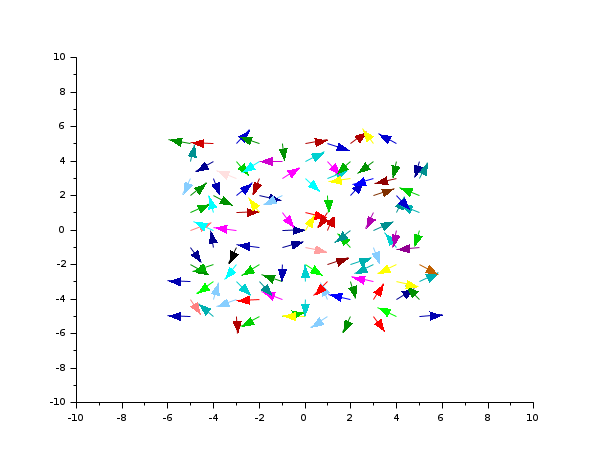Scilab Home page | Wiki | Bug tracker | Forge | Mailing list archives | ATOMS | File exchange
Change language to: English - Português - 日本語 - Русский

See the recommended documentation of this function

Aide de Scilab >> Graphiques > 2d_plot > champ1

# champ1

2D vector field plot with colored arrows

### Syntax

`champ1(x, y, fx, fy, [arfact, rect, strf])`

### Arguments

x, y

two vectors which define the grid.

fx

a matrix which describes the x component of the vector field. `fx(i,j)` is the x component of the vector field at point `(x(i),y(j))`.

fy

a matrix which describes the y component of the vector field. `fy(i,j)` is the y component of the vector field at point `(x(i),y(j))`.

arfact

an optional argument of type `real` which gives a scale factor for the display of the arrow heads on the plot (default value is 1.0).

rect

a vector `rect = [xmin, ymin, xmax, ymax]` which gives the boundaries of the graphics frame to use.

frameflag

controls the computation of the actual coordinate ranges from the minimal requested values. The associated value should be an integer ranging from 0 to 8.

axesflag

specifies how the axes are drawn. The associated value should be an integer ranging from 0 to 5.

strf

a string of length 3 "xyz" which has the same meaning as the `strf` parameter of `plot2d`. The first character `x` has no effect with `champ1`.

### Description

`champ1` draws a 2D vector field with colored arrows. The color of the arrows depends on the intensity of the field.

If you want arrows proportional to the intensity of the field, use `champ`.

Enter the command `champ1()` to see a demo.

Be cautioned about the fact that using meshgrid function before using `champ1` requires adjustment. For more details, see the last example below.

### Examples

`champ1(-5:5,-5:5,rand(11,11),rand(11,11),rect=[-10,-10,10,10],arfact=2)````// using meshgrid to get fx and fy
clf()
x = linspace(-1,1,30);
y = linspace(-1,1,30);
[X,Y] = meshgrid(x,y);
fy = 2.*Y;
fx = 2.*X;
champ1(x,y,fx',fy'); //here we have transposed fx and fy```• champ — 2D vector field plot
• fchamp — direction field of a 2D first order ODE
• plot2d — 2D plot